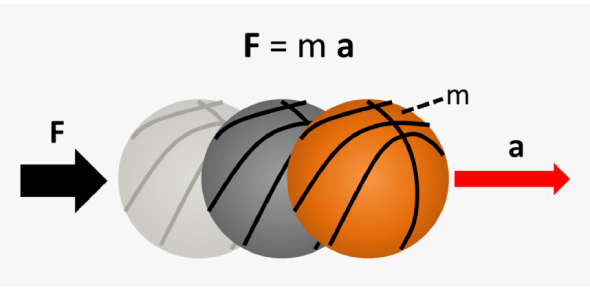# Can You Pass This Physics Quiz On Force? Part II

15 Questions | Total Attempts: 67Settings.

• 1.
No. of unknown internal forces in each member of a rigid jointed plane frame is
• A.

6

• B.

3

• C.

2

• D.

1

• 2.
In plastic analysis, the shape factor for a triangular section, is
• A.

2.34

• B.

1.34

• C.

1.5

• D.

1

• 3.
Pick up the correct statement from the following:
• A.

The ratio of plastic moment to the yield moment, is called shape factor

• B.

In a loaded beam, the moment at which the entire section of the beam becomes fully plastic, is called plastic moment

• C.

In a fully plastic stage of the beam, the neutral axis divides the section in two sections of equal area

• D.

All

• 4.
The point of contraflexure is the point where
• A.

B.M. is minimum

• B.

S.F. is zero.

• C.

B.M. changes sign

• D.

B.M. is maximum

• 5.
The forces in the members of simple trusses, may be analysed by
• A.

Method of joints

• B.

Method of sections

• C.

Graphical method

• D.

All

• 6.
The deflection at any point of a perfect frame can be obtained by applying a unit load at the joint in
• A.

Vertical direction

• B.

Horizontal direction

• C.

Inclined direction

• D.

The direction in which the deflection is required

• 7.
The carryover factor in a prismatic member whose far end is fixed
• A.

0

• B.

1/2

• C.

3/4

• D.

1

• 8.
The Castigliano’s second theorem can be used to compute deflections
• A.

In statically determinate structures only

• B.

For any type of structure

• C.

At the point under the load only

• D.

For beams and frames only

• 9.
For a single point load W moving on a symmetrical three hinged parabolic arch of span L, the maximum sagging moment occurs at distance X from ends. The value of X is
• A.

.211L

• B.

.25 L

• C.

.234 L

• D.

.5 L

• 10.
In the displacement method of structural analysis the basic unknowns are
• A.

Displacements

• B.

Forces

• C.

Displacements and forces

• D.

None of the above

• 11.
For a two hinge arch, if one of the supports  settles down vertically, then the horizontal thrust
• A.

Is increased

• B.

Is decreased

• C.

Remain unchanged

• D.

Becomes zero

• 12.
Which of the following methods of structural analysis is a force method
• A.

Slope deflection Method

• B.

Moment distribution Method

• C.

Column analogy method

• D.

None of the above

• 13.
Rigid jointed plane frame is stable and statically determinate if
• A.

(m+r) = 2j

• B.

(m+r) = 3j

• C.

(3m+r) = 3j

• D.

(m+3r) = 3j

• 14.
When a uniformly distributed load, longer than the span of a girder, moves from left to right, then the conditions of maximum bending moments at a section is that
• A.

• B.

The tail of the load reaches the section

• C.

The load position should be such that the section divides equally on both sides

• D.

The load position should be such that the section divides the load in the same ratio as it divides the span

• 15.
In case of principal axes of a section
• A.

Sum of moment of inertia is zero

• B.

Difference of moment inertia is zero

• C.

Product of moment of inertia is zero

• D.

None of these.

Related TopicsBack to top